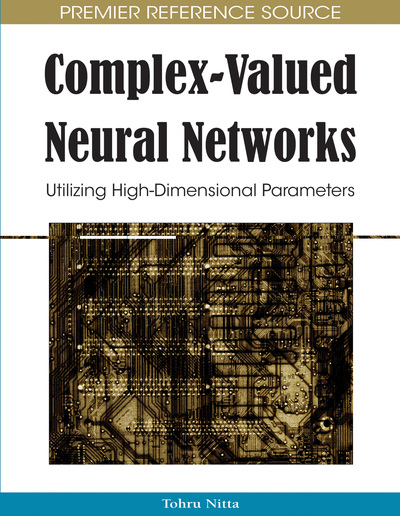# Complex-Valued Symmetric Radial Basis Function Network for Beamforming

Sheng Chen
DOI: 10.4018/978-1-60566-214-5.ch007
OnDemand:
(Individual Chapters)
Available
\$37.50
No Current Special Offers

## Abstract

The complex-valued radial basis function (RBF) network proposed by Chen et al. (1994) has found many applications for processing complex-valued signals, in particular, for communication channel equalization and signal detection. This complex-valued RBF network, like many other existing RBF modeling methods, constitutes a black-box approach that seeks typically a sparse model representation extracted from the training data. Adopting black-box modeling is appropriate, if no a priori information exists regarding the underlying data generating mechanism. However, a fundamental principle in practical data modelling is that if there exists a priori information concerning the system to be modeled it should be incorporated in the modeling process. Many complex-valued signal processing problems, particularly those encountered in communication signal detection, have some inherent symmetric properties. This contribution adopts a grey-box approach to complex-valued RBF modeling and develops a complex-valued symmetric RBF (SRBF) network model. The application of this SRBF network is demonstrated using nonlinear beamforming assisted detection for multiple-antenna aided wireless systems that employ complex-valued modulation schemes. Two training algorithms for this complex-valued SRBF network are proposed. The first method is based on a modified version of the cluster-variation enhanced clustering algorithm, while the second method is derived by modifying the orthogonal-forward-selection procedure based on Fisher ratio of class separability measure. The effectiveness of the proposed complex-valued SRBF network and the efficiency of the two training algorithms are demonstrated in nonlinear beamforming application.
Chapter Preview
Top

## Introducion

The radial basis function (RBF) network is a popular artificial neural network (ANN) architecture that has found wide-ranging applications in many diverse fields of engineering, see for example, (Chen et al., 1990; Leonard & Kramer, 1991; Chen et al., 1993; Caiti & Parisini, 1994; Gorinevsky et al., 1996; Cha & Kassam, 1996; Rosenblum & Davis, 1996; Refaee et al., 1999; Muraki et al., 2001; Mukai, et al., 2002; Su et al., 2002; Li et al., 2004; Lee & Choi, 2004; Ng et al., 2004; Oyang et al., 2005; Acir et al., 2005; Tan et al., 2005). The RBF method is a classical numerical technique for nonlinear functional interpolation with real-valued data (Powell, 1987). A renewed interest in the RBF method coincided with a recent resurgence in the field of ANNs. Connections between the RBF method and the ANN was made and the RBF model was re-interpreted as a one-hidden-layer feedforward network (Broomhead & Lowe, 1988; Poggio & Girosi, 1990). Specifically, by adopting the ANN interpretation, a RBF model can be considered as a processing structure consisting of a hidden layer and an output layer. Each node in the hidden layer has a radially symmetric response around a node parameter vector called a centre, with the hidden node’s response shape determined by the chosen basis function as well as a node width parameter, while the output layer is a set of linear combiners with linear connection weights.

The parameters of the RBF network include its centre vectors and variances or covariance matrices of the basis functions as well as the weights that connect the RBF nodes to the network output. All the parameters of a RBF network can be learned together via nonlinear optimisation using the gradient based algorithms (Chen et al., 1990a; An et al., 1993; McLoone et al., 1998; Karayiannis et al., 2003; Peng et al., 2003), the evolutionary algorithms (Whitehead & Choate, 1994; Whitehead, 1996; Gonzalez et al., 2003) or the expectation-maximisation algorithm (Yang & Chen, 1998; Mak & Kung, 2000). Generally, learning based on such a nonlinear approach is computationally expensive and may encounter the problem of local minima. Additionally, the network structure or the number of RBF nodes has to be determined via other means, typically based on cross validation. Alternatively, clustering algorithms can be applied to find the RBF centre vectors as well as the associated basis function variances (Moody & Darken, 1989; Chen et al., 1992; Chen, 1995; Uykan, 2003). This leaves the RBF weights to be determined by the usual linear least squares solution. Again, the number of the clusters has to be determined via other means, such as cross validation. One of the most popular approaches for constructing RBF networks however is to formulate the problem as a linear learning one by considering the training input data points as candidate RBF centres and employing a common variance for every RBF node. A parsimonious RBF network is then identified using the orthogonal least squares (OLS) algorithm (Chen et al., 1989; Chen et al., 1991; Chen et al., 1999; Chen et al., 2003; Chen et al., 2004a).

## Complete Chapter List

Search this Book:
Reset